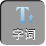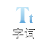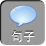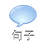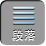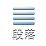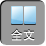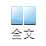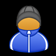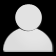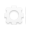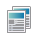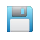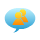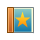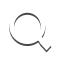-AA+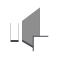1）求 A

2）若 B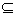A，求实数 a的取值范围.

【考场错解】（ 1）由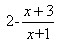0，得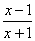0

x< -1x1．即 A=( -∞， -1)∪[ 1，+∞].

2）由( x-a -1)( 2 a-x)＞ 0，得( x-a -1)( x -2 a)< 0

a= 1时， B=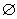，此时 BA

a< 1时， a+ 1> 2 a，∴ B=( 2 aa+ 1),

BA

2 a1a+ 1-1，即 a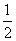a-2

【专家把脉】由函数的概念可知函数的定义域为非空集合，所以错解中 a= 1B=，说明此函数不存在，因此应排除 a= 1.

【对症下药】（ 1）由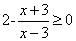，得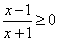x< -1x1．即 A=( -∞， -1)∪[ 1，+∞].

2）由( x-a -1)( 2 a-x)＞ 0，得( x-a -1)( x -2 a)< 0

a= 1时， B=，∵定义域为非空集合，∴ a1

a< 1时， a+ 1> 2 a，∴ B=( 2 aa+ 1),

BA

2 a1a+ 1-1，即 aa-2